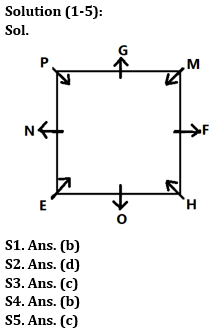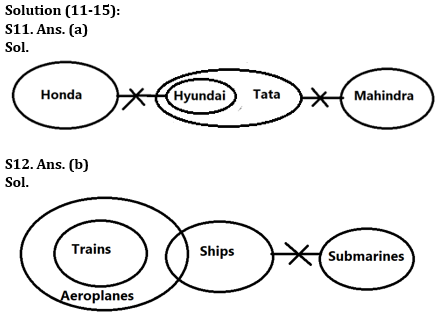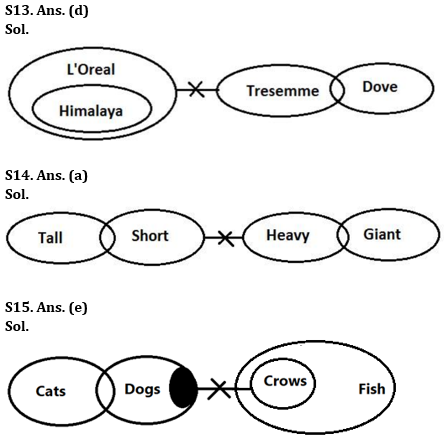Latest Banking jobs   »

# Reasoning Ability Quiz For Bank Foundation 2023 – 27th March

Directions (1-5): Answer the questions based on the information given below.
Eight persons M, N, O, P, E, F, G and H sit around a square table. Four persons sit at the middle of each side while four sit at the corners. Persons at the corner face towards the center while person along the sides face away from the center. (Note: Consecutives named persons neither sit adjacent nor sit opposite to each other).
O sits adjacent to H. M and G are immediate right of each other. H faces towards the center. N sits second to the left of G. E and P face in same direction. F doesn’t face towards the table.

Q1. Who among the following sits opposite to G?
(a) P
(b) O
(c) M
(d) N
(e) F

Q2. How many persons sit between H and G when counted from left of H?
(a) One
(b) Two
(c) Three
(d) Four
(e) None of the above

Q3. Four of the following five are alike in a certain way and hence form a group. Who among the one that doesn’t belong to that group?
(a) O
(b) F
(c) H
(d) G
(e) N

Q4. Who among the following sits second to the right of F?
(a) M
(b) O
(c) N
(d) E
(e) P

Q5. Who among the following faces the same direction as F?
(a) None of these
(b) E
(c) G
(d) M
(e) P

Q6. If in the number 67459138, 3 is subtracted to first five digit of the number and 1 is added to rest of the digits then how many digits are repeating in the number thus formed?
(a) Four
(b) One
(c) None
(d) three
(e) Two

Q7. Which of the following elements should come in a place of ‘?’?
GJ11 MO16 RT21 ?

(a) WX26
(b) VX25
(c) WY26
(d) VY26
(e) None of these

Q8. How many pairs of letters are there in the word “ENDURANCE” each of which have as many letters between them in the word as they have between them in the English alphabetical series?
(a) Two
(b) One
(c) Three
(d) More than three
(e) None

Q9. Ravi is 22nd from the left end of a row and Hunny is 32th from the right end of row. If they interchanged their positions then Ravi ranks become 21 from left end. How many persons sit between them?
(a) One
(b) Two
(c) None
(d) Three
(e) None of these

Q10. Among J, K, L, M and N, each of them is different weight. L’s weight is more than K. M’s weight is more than J and less than N. K is not the lightest person. M is not lighter than L. Who among them is the third heaviest?
(a) J
(b) L
(c) K
(d) M
(e) N

Directions (11-15): In the question below three statements are given followed by two conclusions. You have to take the given statements to be true even if they seem to be at variance with commonly known facts. Read all the conclusions and then decide which of the given conclusions logically follows from the given statements disregarding commonly known facts.

Q11. Statements: No Honda is Hyundai.
All Hyundai are Tata.
No Tata is Mahindra.
Conclusion: I. A few Honda being Tata is possibility
II. No Mahindra is Honda
(a) Only conclusion I follows
(b) Only conclusion II follows
(c) Either conclusion I or II follows
(d) Both conclusions I and II follow
(e) Neither conclusion I nor II follows

Q12. Statements: Only Aeroplanes are trains.
No submarine is ship.
A few Ships are Aeroplanes.
Conclusions: I. A few Ships being Trains is a possibility
II. A few Aeroplanes are not submarines
(a) Only conclusion I follows
(b) Only conclusion II follows
(c) Either conclusion I or II follows
(d) Both conclusions I and II follow
(e) Neither conclusion I nor II follows

Q13. Statements: All Himalaya are L’Oreal.
No L’Oreal is Tresemme.
Only a few Tresemme are Dove.
Conclusions: I. No Himalaya is Tresemme
II. Some Dove are not L’Oreal
(a) Only conclusion I follows
(b) Only conclusion II follows
(c) Either conclusion I or II follows
(d) Both conclusions I and II follow
(e) Neither conclusion I nor II follows

Q14. Statements: Only a few Tall are Short.
No Short is Heavy.
Only a few Heavy are Giant.
Conclusions: I. Some Tall is not Heavy
II. No Giant is Short
(a) Only conclusion I follows
(b) Only conclusion II follows
(c) Either conclusion I or II follows
(d) Both conclusions I and II follow
(e) Neither conclusion I nor II follows

Q15. Statements: Only a few Cats are Dogs.
Some Dogs are not Crows.
All Crows are Fish.
Conclusions: I. Some Dogs are not Fish
II. Some Cats are not Fish
(a) Only conclusion I follows
(b) Only conclusion II follows
(c) Either conclusion I or II follows
(d) Both conclusions I and II follow
(e) Neither conclusion I nor II follows

SolutionsS6. Ans.(e)
Sol. Original number- 67459138
Obtained number- 34126249

S7. Ans.(c)

S8. Ans.(a)

S9. Ans(c)

S10. Ans(b)
Sol.
N > M > L > K > J## FAQs

### What is the selection process of the Bank Clerk?

The selection process of the Bank Clerk is Prelims & Mains.

#### Congratulations!Union Budget 2023-24: Free PDF